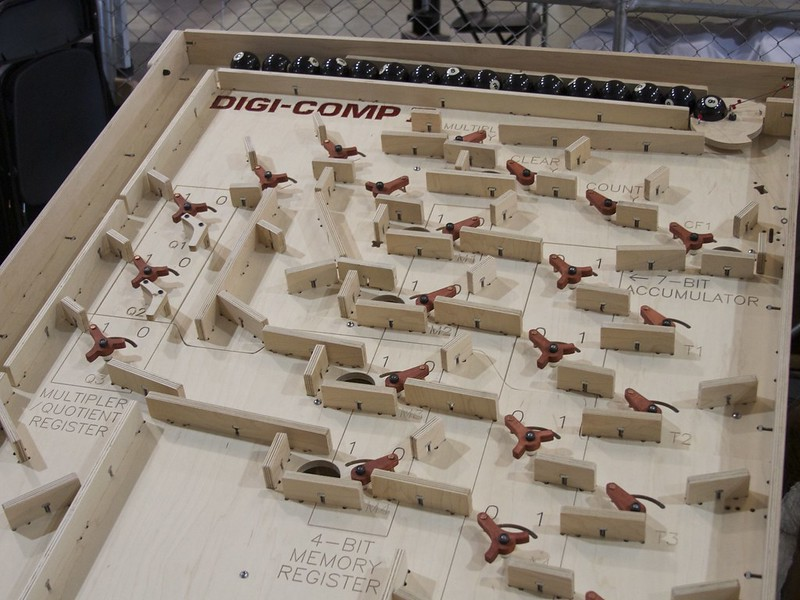Practice on Toph

Participate in exhilarating programming contests, solve unique algorithm and data structure challenges and be a part of an awesome community.

Digi Comp II

Limits 1s, 512 MB

The Digi Comp II is a machine where balls enter from the top and find their way to the bot-tom via a certain circuit defined by switches.Whenever a ball falls on a switch it either goes to the left or to the right depending on the state of the switch and flips this state in the process.Abstractly it can be modelled by a directed graph with a vertex of outdegree2for each switch and in addition a designated end vertex of outdegree0. One of the switch vertices is the start vertex, it has indegree0. Each switch vertex has an internal state (L/R). A ball starts at the start vertex and follows a path down to the end vertex, where at each switch vertex it will pick the left or right outgoing edge based on the internal state of the switch vertex. The internal state of a vertex is flipped after a ball passes through. A ball always goes down and therefore cannot get into a loop.One can “program” this machine by specifying the graph structure, the initial states of each switch vertex and the number of balls that enter. The result of the computation is the state of the switches at the end of the computation. Interestingly one can program quite sophisticated algorithms such as addition, multiplication, division and even the stable marriage problem.However, it is not Turing complete.

Input

The input consists of:

• one line with two integers n (0 ≤ n ≤ 1018) and m (1 ≤ m ≤ 500000), the number of balls and the number of switches of the graph;

• m lines describing switches 1 to m in order. Each line consists of a single character c (‘L’ or ‘R’) and two integers L and R (0 ≤ L, R ≤ m), describing the initial state © of the switch and the destination vertex of the left (L) and right ® outgoing edges. L and R can be equal. Vertex number 0 is the end vertex and vertex 1 is the start vertex. There are no loops in the graph, i.e., after going through a switch a ball can never return to it.

Vertex number 0 is the end vertex and vertex 1 is the start vertex. There are no loops in the graph, i.e., after going through a switch a ball can never return to it.

Output

Output one line with a string of length m consisting of the characters ‘L’ and ‘R’, describing the final state of the switches (1 to min order).

Sample

InputOutput
5 3
L 2 3
R 0 3
L 0 0
RLL

You can find the original problem on the NWERC website.

The photograph is by oskay from Flickr, CC BY-SA.

Discussion
Statistics

NaN% Solution Ratio

Submit